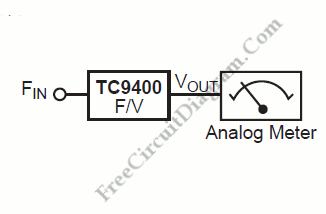# TC9400 Analog Frequency MeterAny frequency below 100kHz will be converted into an output voltage by TC9400 frequency-to-voltage converter. The TC9400 is linear to the input frequency. The equivalent frequency will be displayed on an analog meter. Here is the block diagram of the system:A frequency divider in front of the TC9400 can be used to scale the frequency down into the 100kHz region if the becoming frequency is above 100kHz. [System’s block diagram source: Microchip Application Note]